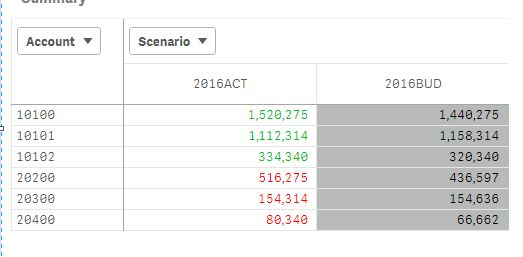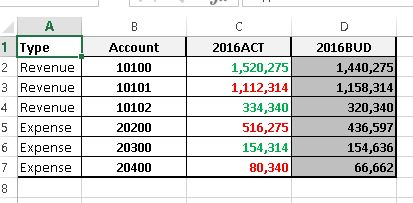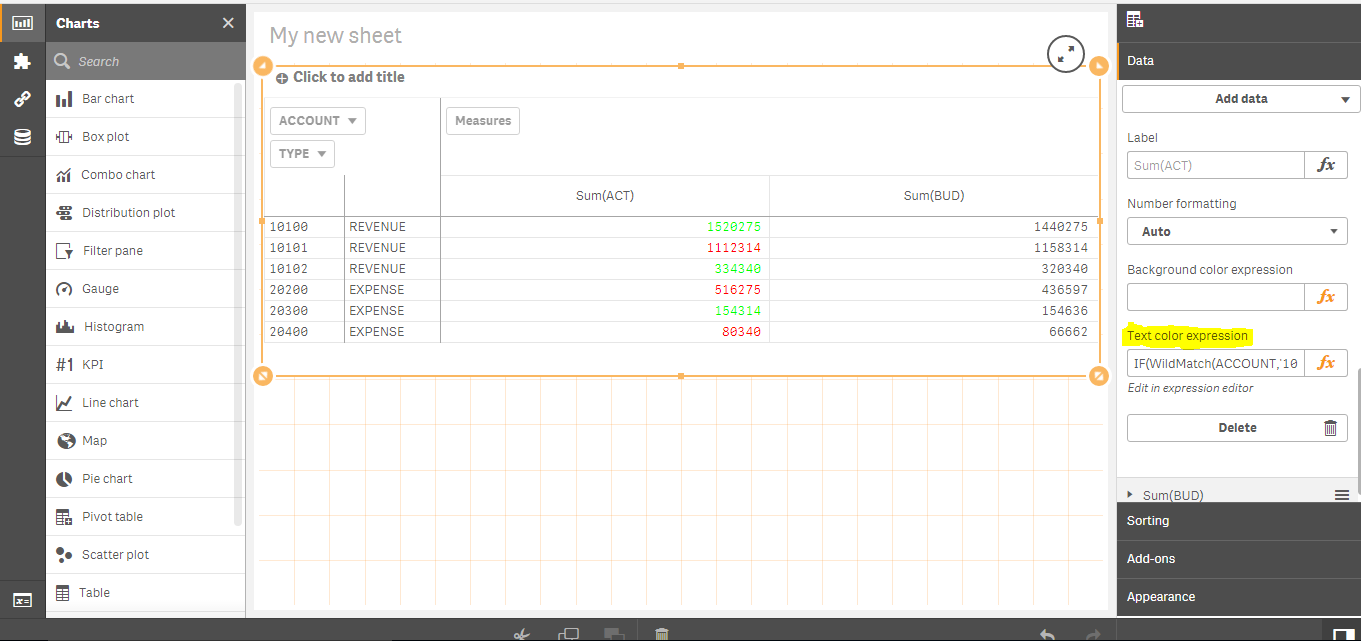# New to Qlik Sense

Discussion board where members can get started with Qlik Sense.

Announcements
Make your voice heard! Participate in the 2020 Wisdom of Crowds® Survey. BEGIN SURVEY
HighlightedPartner

## Text Color Expression of a pivot with Coloring logics

Hi,

In my app, I want to set color for my Actual values using "Text Color expression" in a pivot table. In the attached application I want to apply color for Actual scenarios when my Scenario is always having ACT(Actuals). The color for the values denotes a comparison with Actual and Budget values.

Two types of accounts are there in my application (Revenue and Expense). All Revenue accounts are starting with '101' and all others are expense.

Requirement

1. When actual revenue is more than Budget then it should be in Green Color and if actual Revenue is less than Budget then Red color.

2. When actual expense is more than Budget then it should be in Red color and if actual expense is less than Budget then Green color.

I used the expression in the Text Color Expression field of pivot for this,but its not giving my expected result.

Current Result is shown below.Also attaching my expected result below (screenshot from excel)1 Solution

Accepted Solutions
HighlightedPartner

## Re: Text Color Expression of a pivot with Coloring logics

Have a look at attached app.

7 Replies
HighlightedPartner

## Re: Text Color Expression of a pivot with Coloring logics

Maybe:sthing like

if(Account like "101*" and sum(Actual) >= sum(Budget), green(),

if(Account like "101*" and sum(Actual) < sum(Budget), red(),

if(Account like "20*" and sum(Actual) >= sum(Budget), red(),

if(Account like "20*" and sum(Actual) < sum(Budget), green()

))))

Highlighted
Valued Contributor II

## Re: Text Color Expression of a pivot with Coloring logics

You may want to try similar to following with your required set analysis. As your QVF file doesn't have any data I couldn' test it.

If (AcctType='Revenue',

If(Sum( {<Set Analysis>}     Actual) >= Sum(  {<Set Analysis>}   Budget), RGB(0,255,0), RGB(255,0,0)),

If(Sum(  {<Set Analysis>}    Actual) <= Sum(  {<Set Analysis>}  Budget), RGB(0,255,0), RGB(255,0,0)))

HighlightedPartner

## Re: Text Color Expression of a pivot with Coloring logics

App you attached is giving zero values in 2016ACT & 2016BUD

HighlightedPartner

## Re: Text Color Expression of a pivot with Coloring logics

Have a look at attached app.

Highlighted
Contributor II

## Re: Text Color Expression of a pivot with Coloring logicsTEXT COLOR EXPRESSION:

IF(WildMatch(ACCOUNT,'101*') AND SUM(ACT)>=SUM(BUD),RGB(0,255,0),

IF(WildMatch(ACCOUNT,'101*') AND SUM(ACT)<SUM(BUD),RGB(255,0,0),

IF(WildMatch(ACCOUNT,'20*') AND SUM(ACT)>=SUM(BUD),RGB(255,0,0),

IF(WildMatch(ACCOUNT,'20*') AND SUM(ACT)<SUM(BUD),RGB(0,255,0),

))))

HighlightedPartner

## Re: Text Color Expression of a pivot with Coloring logics

This fits in my exact requirement.

Really AppreciatedRegards

BijoyPartner